Box Plots MathBitsNotebook.com Terms of Use   Contact Person: Donna Roberts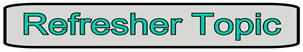Often times, we need to know more about our data than just a possible center value.
One of the additional pieces of information that we may need is the actual distribution of the data (how the data is spread out). To find this information, we examine the data for a
five statistical summary (or five number summary): (1) minimum, (2) maximum, (3) median (second quartile), (4) first quartile, and (5) third quartile. These pieces of information will show the extent to which the data is located near the center or near the extremes of the set.Before we look further at a five statistical summary, let's refresh our skills on quartiles.Quartiles

A median divides a data set into two equal parts. The set can be subdivide further into four equal parts, by values called quartiles. The quartiles divide the data set into quarters, with each quarter containing one-fourth (or 25%) of the data. The quartiles are like additional "medians" of the lower and upper halfs of the data set. A quartile is a number, it is not a range of values. Data can be described as being "above" or "below" the first quartile, but data is never "in" the first quartile.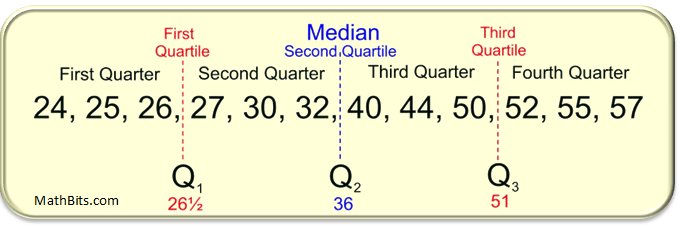Q1: The first quartile is the middle (the median) of the lower half of the data set. One-fourth (25%) of the data lies below the first quartile, and three-fourths (75%) lies above. Q2: The second quartile is another name for the median of the entire set. One-half (50%) of the data lies below the second quartile, and one-half (50%) lies above. Q3: The third quartile is the middle (the median) of the upper half of the data set. Three-fourths (75%) of the data lies below the third quartile and one-fourth (25%) lies above.

The difference between the third quartile and first quartile is called
the interquartile range (IQR).
The interquartile range (also called the midspread or middle fifty), is the distance between the third and first quartiles and is considered a more stable statistic than the "range" of the set.
The IQR contains 50% of the data.

 IQR = Q3 - Q1
For the example shown above, the IQR = 51 - 26½ = 24½.

It may be the case that a data value falls well outside the range of the other values in the set. Such data values are called outliers (as they "lie outside" the other values) . We will see, later on this page, that outliers may lead to false impressions regarding the distribution of a data set.

Outliers are defined as those data points that fall more than a specified distance from the first or third quartiles. That specified distance is 1.5 • IQR (one and one-half times the IQR). Data points that fall to the far left, or far right, of an ordered data set should be tested as possible outliers.
 Outliers are: greater than Q3 + (1.5 • IQR) (referred to as the upper fence) or less than Q1 - (1.5 • IQR) (referred to as the lower fence)Five Statistical Summary

Let's describe our data set (discussed above) with a five statistical summary:
minimum, maximum, median, first quartile
and third quartile.
DATA SET: {24, 25, 26, 27, 30, 32, 40, 44, 50, 52, 55, 57}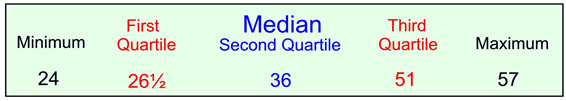While not telling every value in the data set,
a
five statistical summary will tell you that:
• half (50%) of the data values are below 36,
• half (50%) of the data values are above 36, and
• half (50%) of the scores are between 26½ and 51.
It also tells how the data break out in quarters,
along with the smallest and largest data values.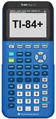For calculator help with five-number summary click here.Box & Whiskers
A five statistical summary can be represented graphically as a box and whisker plot (or box plot). The first and third quartiles are the ends of the box, the median is indicated with a vertical line in the interior of the box, and the minimum and maximum are the ends of the whiskers (unless an outlier is present). Each of the four "sections" of a box plot represents 25% of the data in the set.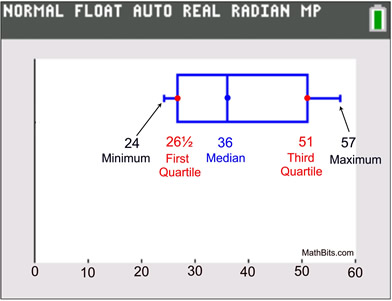How to construct a box and whisker plot by hand:

 Write the data in ascending numerical order. Find the minimum, first quartile, median, third quartile and maximum (the five statistical summary). minimum = 24 first quartile = 26½ median = 36 third quartile = 51 maximum = 57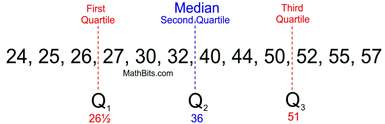Prepare an equally spaced number line that will contain your values. Place a large dot beneath each of the five statistical summary values on the number line. You may place the dots ON the line or BELOW the line.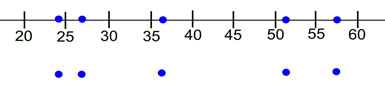Draw a box with the ends through the points for the first and third quartiles. Draw a vertical line through the box at the median. Draw the whiskers from each end of the box to the minimum and maximum values (unless you have an outlier).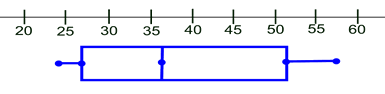Note: While box and whisker plots are generally drawn horizontally (as shown above), it is also acceptable to draw box and whisker plots vertically.

 So what do you do if you have an outlier? Data Set: {1, 30, 40, 44, 44, 44, 45, 46, 47, 51, 54, 54, 55} It certainly looks like the "1" is not in keeping with the rest of these values. Let's test it to see if it is an outlier. First, we need to find the first and third quartiles: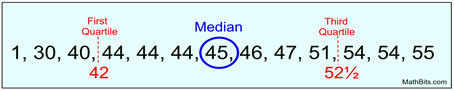Now, do the calculations to test for an outlier: Is "1" less than Q1 - (1.5 • IQR)?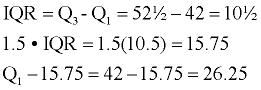Since "1" is less than 26.25, "1" is definitely an outlier. The "1" is plotted as a single dot (or asterisk *), separate from the box's whisker . The whisker then uses 30 as its minimum point.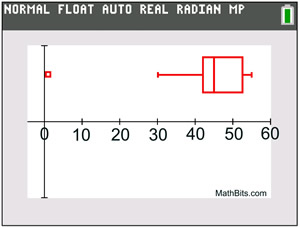Graph with outlier. If this outlier is used as the end point of the left whisker, readers may think that there are grades dispersed evenly throughout the whole range from 1 to 42, which is not the case. The use of the separately plotted outlier gives us more reliable information about this data set.Did you notice that the IQR is actually the horizontal length of the box in a box and whisker plot? Thus, an outlier is any value that lies more than one and one-half times the length of the box from either end of the box.For calculator help with box and whisker plots click here.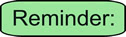Box plots: Pros and Cons While a box and whisker plot displays several important features of a distribution, it does not show the distribution of the data in as much detail as a histogram or dot plot. Box plots are useful, however, for quickly indicating whether the distributions are skewed, and whether there are any outliers in the data set. Box plots are also useful when representing large amounts of data, and when comparing data sets.

Box plots show the shape of the distribution of the data, the central value, and the variability.
It uses the median as its center value and presents a brief picture of the distribution of the other values in the form of its five statistical summary.Shapes of Plots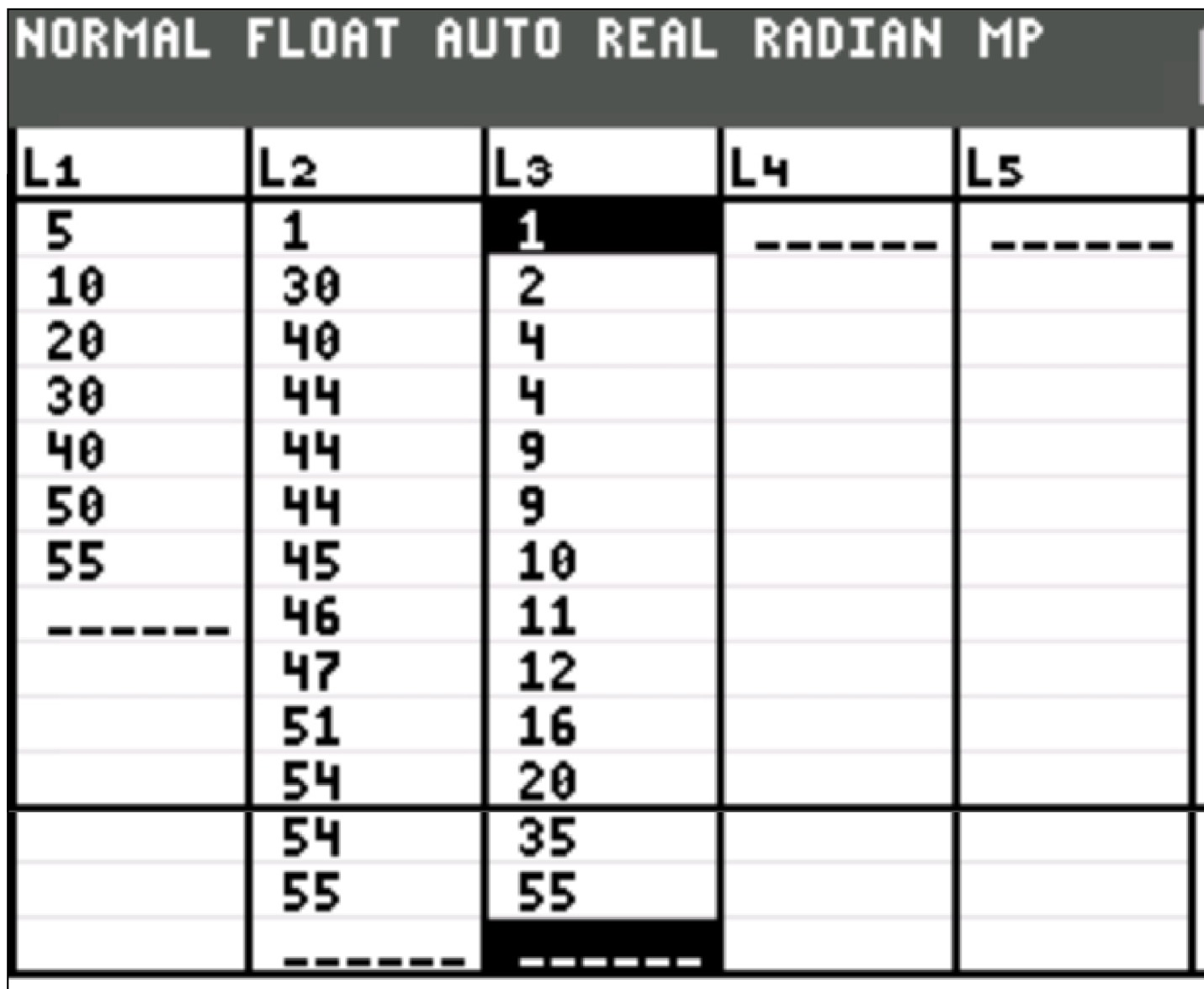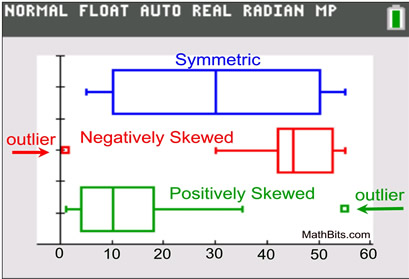Symmetric:
If a box and whisker plot is symmetric, the median is equidistant from the minimum and the maximum.

Neatively Skewed: If a box and whisker plot is negatively skewed, the distance from the median to the minimum is greater than the distance from the median to the maximum.

Positively Skewed: If a box and whisker plot is positively skewed, the distance from the median to the maximum is greater than the distance from the median to the minimum.For calculator help with box and whisker plots click here.For calculator help with five-number summary click here.